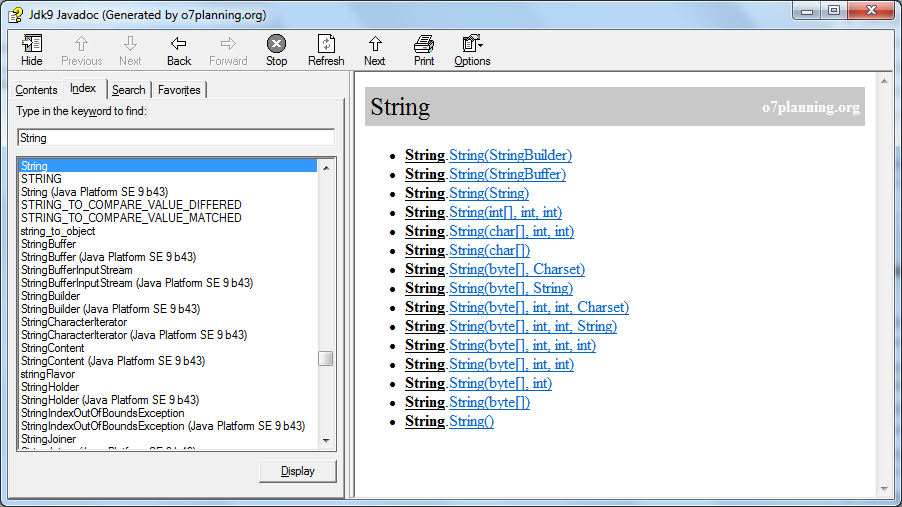# Quick Learning Java for beginners

#### View more Tutorials: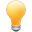Follow us on our fanpages to receive notifications every time there are new articles.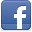Facebook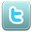Twitter

## 1- Introduction

First of all, you should see this document:

## 2- Create new Project

First we create a new Project, it will be used in this tutorial.Enter the name of the project:
• BasicJavaTutorial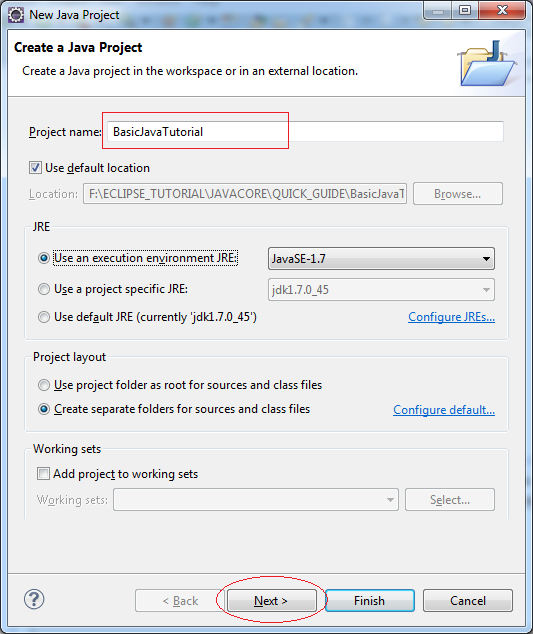Project has been created: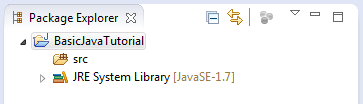Note: To be able to write in languages other than English in the Project, you should switch to UTF8 encoding.
Right-click the Project and select Properties: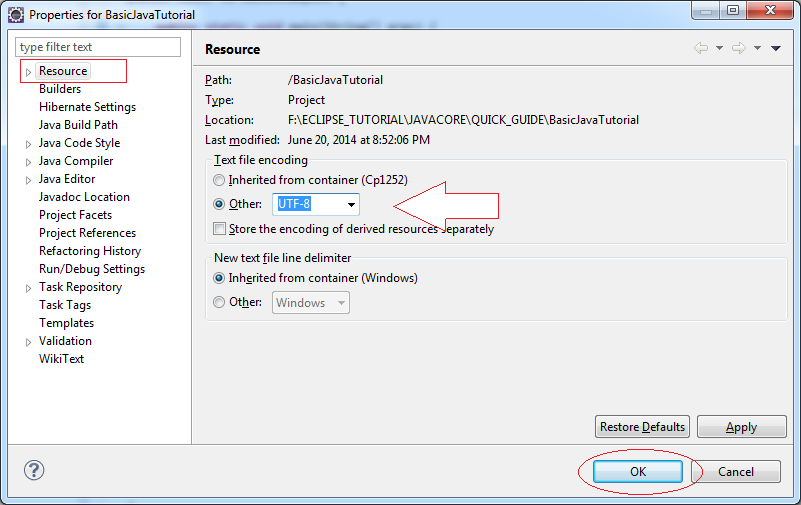## 3- Primitive Data Types

There are eight primitive types in JAVA
• For integer has 4 types: byte, short, int, long
• Real data type we have: float, double
• Character type: char
• Boolean: returns true or false value (true or false)
 Type Description bit Min value Max value byte 8 bit integer number 8 -128 (-2^7) 127 (2^7-1) short 16 bit integer number 16 -32,768 (-2^15) 32,767 (2^15 -1) int 32 bit integer number 32 - 2,147,483,648 (-2^31) 2,147,483,647 (2^31 -1) long 64 bit integer number 64 -9,223,372,036,854,775,808 (-2^63) 9,223,372,036,854,775,807 (2^63 -1) float 32 bit real number 32 -3.4028235 x 10^38 3.4028235 x 10^38 double 64 bit real number 64 -1.7976931348623157 x 10^308 1.7976931348623157 x 10^308 boolean Logic type false true char Character type 16 '\u0000' (0) '\uffff' (65,535).
See more details:

## 4- Variable

Right-click the src select "New/Package":Name the package are:
• org.o7planning.tutorial.javabasic.variable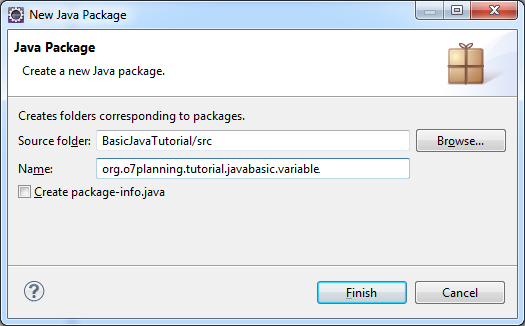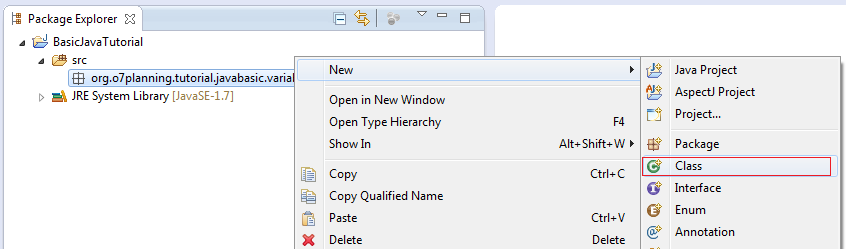Enter the name of the class:
• VariableExample1VariableExample1 class is created: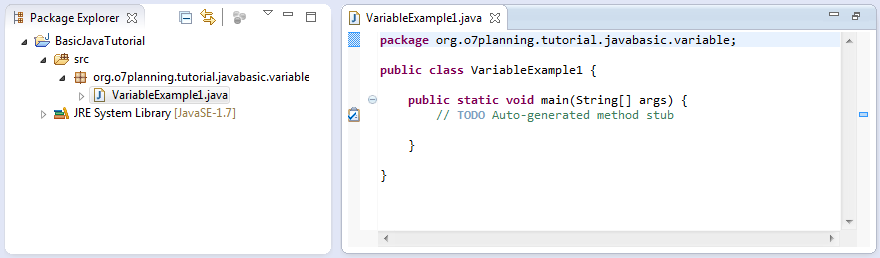Edit VariableExample1 class:
VariableExample1.java
``````
package org.o7planning.tutorial.javabasic.variable;

public class VariableExample1 {

public static void main(String[] args) {

// Declare a variable of type int (32bit Integer)
int firstNumber;

// Assigning value to firstNumber
firstNumber = 10;

System.out.println("First Number =" + firstNumber);

// Declare a variable type of float (32-bit real number)
// This number is assigned a value of 10.2
// The 'f' character at the end makes java understand that this is a float.
float secondNumber = 10.2f;

System.out.println("Second Number =" + secondNumber);

// Declare a number type of double (64-bit real number).
// This number is assigned a value of 10.2
// Character 'd' at the end to tell with Java this is the type double.
// Distinguished from a float 'f'.
double thirdNumber = 10.2d;

System.out.println("Third Number =" + thirdNumber);

// Declare a character
char ch = 'a';

System.out.println("Char ch= " + ch);

}

}
``````
Run class VariableExample1:
Right-click on the class VariableExample1 select "Run As/Java Application":Results of running the class to see on the Console: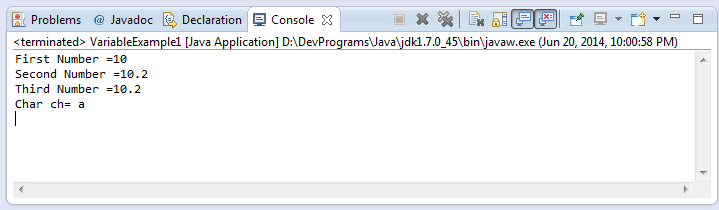You can also declare multiple variables at once, the next example illustrates this:
Create a new class VariableExample2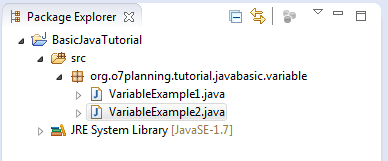VariableExample2.java
``````
package org.o7planning.tutorial.javabasic.variable;

public class VariableExample2 {

public static void main(String[] args) {

// Declare 3 numbers type of long (64-bit Integer)
long firstNumber, secondNumber, thirdNumber;

// Assign value to firstNumber
// Character 'L' at the end to tell java a long type,
// distinguished from type int.
firstNumber = 100L;

// Assign values to secondNumber
secondNumber = 200L;

// Assign values to thirdNumber
thirdNumber = firstNumber + secondNumber;

System.out.println("First Number = " + firstNumber);
System.out.println("Second Number = " + secondNumber);
System.out.println("Third Number = " + thirdNumber);
}

}
``````
Results of running the class VariableExample2:
``````
First Number = 100
Second Number = 200
Third Number = 300
``````

## 5- Control flow

### 5.1- if-else statement

The structure of an if-else statement is:
``````
if(condition 1)  {
// Do something here
}else if(condition 2) {
// Do something here
}else if(condition 3) {
// Do something here
}
// Else
else  {
// Do something here
}
``````
Create class ElseIfExample1: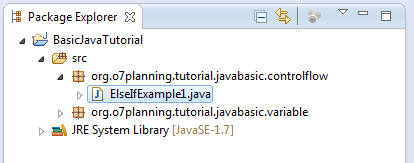ElseIfExample1.java
``````
package org.o7planning.tutorial.javabasic.controlflow;

public class ElseIfExample1 {

public static void main(String[] args) {

// Declaring a varible of type int (32 bit integer)
int score = 20;

// If the score is less than 50
if (score < 50) {
System.out.println("You are not pass");
}
// Else if the score more than or equal to 50 and less than 80.
else if (score >= 50 && score < 80) {
System.out.println("You are pass");
}
// Remaining cases (that is greater than or equal to 80)
else {
System.out.println("You are pass, good student!");
}

}
}
``````
Results of running the class ElseIfExample1:
``````
You are not pass
``````
Change the value of the variable "score" in the above example and rerun the class ElseIfExample1:
``````
int score = 60;
``````
``````
You are pass
``````

### 5.2- The normal operator

• > Greater Than
• < Less Than
• >= Greater Than or Equal To
• <= Less Than or Equal To
• && AND
• || OR
• == HAS A VALUE OF
• != Not Equal To
• ! NOT
Create class ElseIfExample2
ElseIfExample2.java
``````
package org.o7planning.tutorial.javabasic.controlflow;

public class ElseIfExample2 {

public static void main(String[] args) {

// Declare a variable of type int, represents your age.
int age = 20;

// Test if age less than or equal 17
if (age <= 17) {
System.out.println("You are 17 or younger");
}

// Test age equals 18
else if (age == 18) {
System.out.println("You are 18 year old");
}
// Test if age greater than 18 and less than 40
else if (age > 18 && age < 40) {
System.out.println("You are between 19 and 39");
}
// Remaining cases (Greater than or equal to 40)
else {
// Nested if statements
// Test age not equals 50.
if (age != 50) {
System.out.println("You are not 50 year old");
}

// Negative statements
if (!(age == 50)) {
System.out.println("You are not 50 year old");
}

// If age is 60 or 70
if (age == 60 || age == 70) {
System.out.println("You are 60 or 70 year old");
}

}

}
}
``````
You can change the value of "age" and rerun ElseIfExample2 class and see the results

### 5.3- Boolean value

boolean is a data type, it only has two values true or false.
Create class BooleanExample: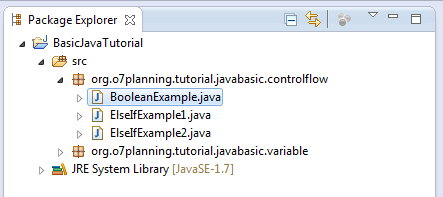BooleanExample.java
``````
package org.o7planning.tutorial.javabasic.controlflow;

public class BooleanExample {

public static void main(String[] args) {

// Declare a variable of type boolean
boolean value = true;

// If value is true
if (value == true) {
System.out.println("It's true");
}
// Else
else {
System.out.println("It's false");
}

if (value) {
System.out.println("It's true");
}
// Else
else {
System.out.println("It's false");
}
}
}
``````

### 5.4- switch- case -default statement

This is also a branch statement similar to the if-else is introduced above
``````
// variable_to_test: A varible to test.
switch ( variable_to_test ) {
case value1:
// Do something here ...
break;
case value2:
// Do something here ...
break;
default:
// Do something here ...
}
``````
SwitchExample1.java
``````
package org.o7planning.tutorial.javabasic.controlflow;

public class SwitchExample1 {

public static void main(String[] args) {

// Declare a variable age
int age = 20;

// Check the value of age
switch (age) {
// Case age = 18
case 18:
System.out.println("You are 18 year old");
break;
// Case age = 20
case 20:
System.out.println("You are 20 year old");
break;
// Remaining cases
default:
System.out.println("You are not 18 or 20 year old");
}

}

}
``````
The results of running the class SwitchExample1:
``````
You are 20 year old
``````
Note that the case statement is a specific value you can not do the following:
``````
// This is not allowed !!
case (age < 18) :

// case only accept a specific value, eg:
case 18:
// Do something here ..
break;
``````
See an example:
SwitchExample2.java
``````
package org.o7planning.tutorial.javabasic.controlflow;

public class SwitchExample2 {

public static void main(String[] args) {

// Declare a variable age.
int age = 30;

// Check the value of age.
switch (age) {
// Case age = 18
case 18:
System.out.println("You are 18 year old");
break;
// Case age in 20, 30, 40
case 20:
case 30:
case 40:
System.out.println("You are " + age);
break;
// Remaining case:
default:
System.out.println("Other age");
}

}

}
``````
The results run:
``````
You are 30
``````

### 5.5- for Loop

Syntax:
``````
// start_value: Start Value
// end_value: End Value
// increment_number: Added value after each iteration.
for ( start_value; end_value; increment_number ) {
// Do something here
}
``````
Consider an illustration: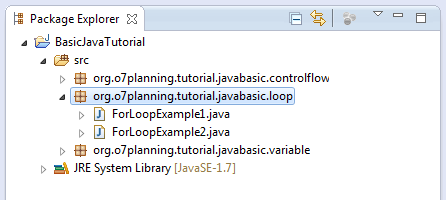ForLoopExample1.java
``````
package org.o7planning.tutorial.javabasic.loop;

public class ForLoopExample1 {

public static void main(String[] args) {

// Declare a variable 'step', step in loop
int step = 1;

// Declare a variable value with the start value is 0
// After each iteration, value will increase 3
// And the loop will end when the value greater than or equal to 10
for (int value = 0; value < 10; value = value + 3) {

System.out.println("Step =" + step + "  value = " + value);

// Increase 1
step = step + 1;

}

}

}
``````
Results of running the class ForLoopExample1:
``````
Step =1  value = 0
Step =2  value = 3
Step =3  value = 6
Step =4  value = 9
``````
See another example, sum the numbers from 1 to 100:
ForLoopExample2.java
``````
package org.o7planning.tutorial.javabasic.loop;

public class ForLoopExample2 {

// This is an example to sum the numbers from 1 to 100,
// and print out the results to the console.
public static void main(String[] args) {

// Declare a variable
int total = 0;

// Declare a variable value
// Initial value is 1
// After each iteration increases the 'value' by adding 1
// Note: value++ equivalent to the statement: value = value + 1;
// value-- equivalent to the statement: value = value - 1;
for (int value = 1; value <= 100; value++) {

// Increase 'total' by adding 'value'.
total = total + value;
}

System.out.println("Total = " + total);

}

}
``````
Result:
``````
Total = 5050
``````

### 5.6- while Loop

This is while loop structure:
``````
// While condition is true then do something.
while ( condition ) {
// Do something here...
}
``````
See illustration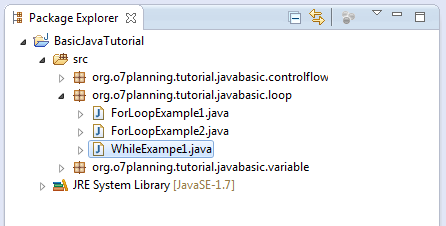WhileExample1.java
``````
package org.o7planning.tutorial.javabasic.loop;

public class WhileExampe1 {

public static void main(String[] args) {

int value = 3;

// While the 'value' is still less than 10, the loop still works.
while (value < 10) {

System.out.println("Value = " + value);

// Increase 'value' by adding 2
value = value + 2;
}
}

}
``````
``````
Value = 3
Value = 5
Value = 7
Value = 9
``````

### 5.7- do-while Loop

This is the structure do-while loop:
``````
// The do-while loop performs at least one iteration.
// And while the condition is true, it continues to work
do {
// Do something here.
}
while (condition);
``````
Example: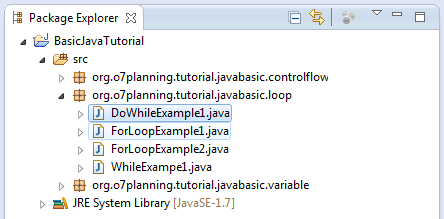DoWhileExample1.java
``````
package org.o7planning.tutorial.javabasic.loop;

public class DoWhileExample1 {

public static void main(String[] args) {

int value = 3;

// do-while loop will execute at least once
do {

System.out.println("Value = " + value);

// Increase by 3
value = value + 3;

} while (value < 10);

}
}
``````
Result:
``````
Value = 3
Value = 6
Value = 9
``````

## 6- Array in Java

### 6.1- What is Array?

An array is a list of the elements are arranged adjacent to each other in memory.
Let's see, a array with 5 elements, int type.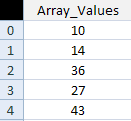### 6.2- Working with arrays

How to declare an array in Java.
``````
// Declare an array, not a specified number of elements.
int[] array1;

// Initialize the array with 100 elements
// The elements has not been assigned a specific value
array1 = new int;

// Declare an array specifies the number of elements
// The elements has not been assigned a specific value
double[] array2 = new double;

// Declare an array with elements assigned a specific values.
// This array has 4 elements
long[] array3= {10L, 23L, 30L, 11L};
``````
Let's look at an example: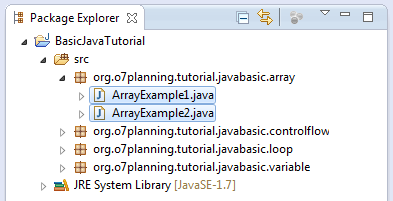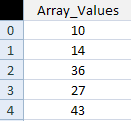ArrayExample1.java
``````
package org.o7planning.tutorial.javabasic.array;

public class ArrayExample1 {

public static void main(String[] args) {

// Declare an array with 5 elements
int[] myArray = new int;

// Note: the first element of the array index is 0:
// Assigning values to the first element (index 0)
myArray = 10;

// Assigning value to the second element (index 1)
myArray = 14;

myArray = 36;
myArray = 27;

// Assigning value to the 5th element (the last element in the array)
myArray = 18;

// Print out element count.
System.out.println("Array Length=" + myArray.length);

// Print to Console element at index 3 (4th element in the array)
System.out.println("myArray=" + myArray);

// Use a for loop to print out the elements in the array.
for (int index = 0; index < myArray.length; index++) {
System.out.println("Element " + index + " = " + myArray[index]);
}
}

}
``````
Result:
``````
Array Length=5
myArray=27
Element 0 = 10
Element 1 = 14
Element 2 = 36
Element 3 = 27
Element 4 = 18
``````
An illustrative example to use a for loop to assign values to the elements:
ArrayExample2.java
``````
package org.o7planning.tutorial.javabasic.array;

public class ArrayExample2 {

public static void main(String[] args) {

// Declare an array with 5 elements
int[] myArray = new int;

// Print out element count
System.out.println("Array Length=" + myArray.length);

// Using loop assign values to elements of the array.
for (int index = 0; index < myArray.length; index++) {
myArray[index] = 100 * index * index + 3;
}

// Print out the element at index 3
System.out.println("myArray = " + myArray);
}

}
``````
Result:
``````
Array Length=5
myArray = 903
``````

## 7- Class, Instance, Constructor

You need to have a distinction between three concepts:
• Class
• Constructor
• Instance
When we talk about the tree, it is something abstract, it is a class. But when we pointed to a specific tree, it was clear, and that is the instance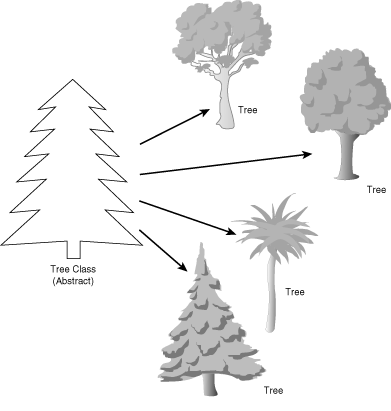Or when we talk about the Person, that's abstract, it is a class. But when pointed at you or me, it is two different instances, the same class of People.Person.java
``````
package org.o7planning.tutorial.javabasic.javastructure;

public class Person {

// This is field
// The name of Person
public String name;

// This is a Constructor
// Use it to initialize the object (Create new object)
// This constructor has one parameter
// The constructor always has the same name as the class name.
public Person(String persionName) {
// Assign the value of the parameter into the 'name' field.
this.name = persionName;
}

// This method returns a String ..
public String getName() {
return this.name;
}

}
``````
Person class has no main method. TestPerson class is initialized instance of the Person object via the constructor
PersonTest.java
``````
package org.o7planning.tutorial.javabasic.javastructure;

public class PersonTest {

public static void main(String[] args) {

// Create an object of class Person
// Initialize this object via constructor of class Person
Person edison = new Person("Edison");

// Class Person has the getName() method.
// Use the object to call getName():
String name = edison.getName();
System.out.println("Person 1: " + name);

// Create an object of class Person
// Initialize this object via constructor of class Person
Person billGate = new Person("Bill Gates");

// Class Person has field name, that is public.
// Use objects to refer to it.
String name2 = billGate.name;
System.out.println("Person 2: " + name2);

}

}
``````
Results of running the example:
``````
Person 1: Edison
Person 2: Bill Gates
``````

## 8- Field

In this section we will discuss some of the concepts:
Field
• Normal field
• static Field
• final Field
• static final Field
See an example with the field and static fields.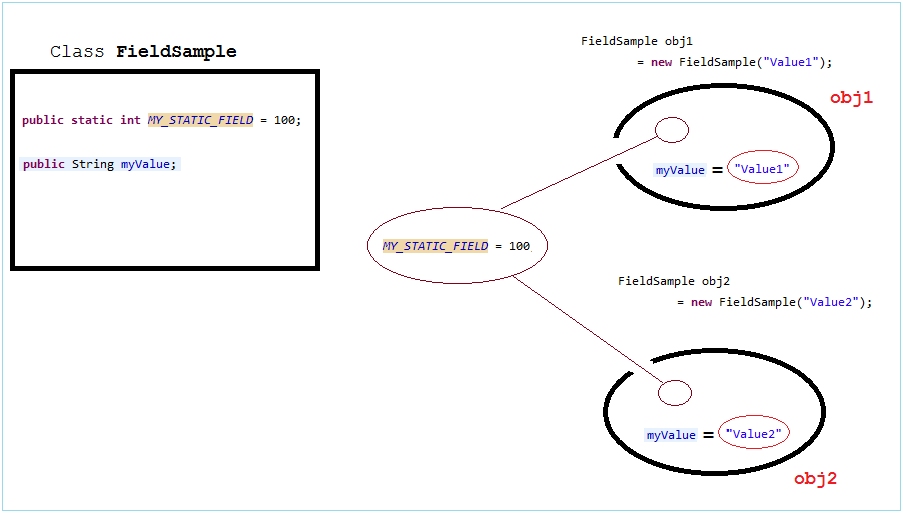FieldSample.java
``````
package org.o7planning.tutorial.javabasic.javastructure;

public class FieldSample {

// This is static field.
public static int MY_STATIC_FIELD = 100;

// This is normal field.
public String myValue;

// Constructor to initialize the FieldSample object.
public FieldSample(String myValue) {
this.myValue = myValue;
}

}
``````
FieldSampleTest.java
``````
package org.o7planning.tutorial.javabasic.javastructure;

public class FieldSampleTest {

public static void main(String[] args) {

// Create the first object.
FieldSample obj1 = new FieldSample("Value1");

System.out.println("obj1.myValue= " + obj1.myValue);

// Print out static value - MY_STATIC_FIELD
// Access via instance of class (an object)(Not recommended)
System.out.println("obj1.MY_STATIC_FIELD= " + obj1.MY_STATIC_FIELD);

// Print out static value - MY_STATIC_FIELD
// Access via class (Recommended)
System.out.println("FieldSample.MY_STATIC_FIELD= " + FieldSample.MY_STATIC_FIELD);

// Create second object:
FieldSample obj2 = new FieldSample("Value2");

System.out.println("obj2.myValue= " + obj2.myValue);

System.out.println("obj2.MY_STATIC_FIELD= " + obj2.MY_STATIC_FIELD);

System.out.println(" ------------- ");

// Set new value for static field.
// (Or using: FieldSample.MY_STATIC_FIELD = 200)
obj1.MY_STATIC_FIELD = 200;

// It will print out the value 200.
System.out.println("obj2.MY_STATIC_FIELD= " + obj2.MY_STATIC_FIELD);
}

}
``````
Results of running the example:
``````
obj1.myValue= Value1
obj1.MY_STATIC_FIELD= 100
FieldSample.MY_STATIC_FIELD= 100
obj2.myValue= Value2
obj2.MY_STATIC_FIELD= 100
-------------
obj2.MY_STATIC_FIELD= 200
``````
The final field is the field that can not assign a new value to it, it's like a constant.
FinalFieldExample.java
``````
package org.o7planning.tutorial.javabasic.javastructure;

public class FinalFieldExample {

// A final field.
// Final Field does not allow to assign new values.
public final int myValue = 100;

// A static final field.
// Final field does not allow to assign new values.
public static final long MY_LONG_VALUE = 1234L;

}
``````

## 9- Method

Method
• Method.
• static Method
• final Method. (Will be mentioned in the inheritance of the class).
MethodSample.java
``````
package org.o7planning.tutorial.javabasic.javastructure;

public class MethodSample {

public String text = "Some text";

// Default Constructor
public MethodSample() {

}

// This method return a String.
// And has no parameter.
public String getText() {
return this.text;
}

// This is a method with one parameter String.
// This method returns void (not return anything)
public void setText(String text) {
// this.text reference to the text field.
// Distinguish the text parameter.
this.text = text;
}

// This is a static method.
// return type of int, has 3 parameters.
public static int sum(int a, int b, int c) {
int d = a + b + c;
return d;
}
}
``````
MethodSampleTest.java
``````
package org.o7planning.tutorial.javabasic.javastructure;

public class MethodSampleTest {

public static void main(String[] args) {

// Create instance of MethodSample
MethodSample obj = new MethodSample();

// Call getText() method
String text = obj.getText();

System.out.println("Text = " + text);

// Call setText(String) method
obj.setText("New Text");

System.out.println("Text = " + obj.getText());

// Static method can be called through the class.
// This way is recommended. (**)
int sum = MethodSample.sum(10, 20, 30);

System.out.println("Sum  10,20,30= " + sum);

// Or call through object.
// This way is not recommended. (**)
int sum2 = obj.sum(20, 30, 40);

System.out.println("Sum  20,30,40= " + sum2);
}

}
``````
``````
Text = Some text
Text = New Text
Sum  10,20,30= 60
Sum  20,30,40= 90
``````

## 10- Inheritance in Java

Java allows classes which extend from other class. Class extends another class called subclasses. Subclasses have the ability to inherit the fields and methods from the parent class.
Animal.java
``````
package org.o7planning.tutorial.javabasic.inheritance;

public class Animal {

public Animal() {

}

public void move() {
System.out.println("Move ...!");
}

// The cry of this animal.
public void say() {
System.out.println("<nothing>");
}

}
``````
Cat.java
``````
package org.o7planning.tutorial.javabasic.inheritance;

public class Cat extends Animal {

// Override method of the Animal class.
public void say() {
System.out.println("Meo");
}

}
``````
Dog.java
``````
package org.o7planning.tutorial.javabasic.inheritance;

public class Dog extends Animal {

// Override method of the Animal class.
public void say() {
System.out.println("Go");
}

}
``````
Ant.java
``````
package org.o7planning.tutorial.javabasic.inheritance;

// Ant
public class Ant extends Animal {

}
``````
AnimalTest.java
``````
package org.o7planning.tutorial.javabasic.inheritance;

public class AnimalTest {

public static void main(String[] args) {

// Declaring a Cat object.
Cat cat = new Cat();

// Check 'cat' instance of Animal.
// The result is clearly true.
boolean isAnimal = cat instanceof Animal;
System.out.println("cat instanceof Animal?" + isAnimal);

// ==> Meo
// Call the method say() of the Cat.
cat.say();

// Declare an object of type Animal
// Initialize the object through the Constructor of the Cat.
Animal cat2 = new Cat();

// ==> Meo
// say() method of Cat is called (Not Animal)
cat2.say();

// Create the object Animal.
// Through the Constructor of the subclass, Ant.
Animal ant = new Ant();

// Ant has no say() method.
// ==> It call to say() method,
// that inherited from the parent class (Animal)
ant.say();
}
}
``````
Results of running the example:
``````
cat instanceof Animal?true
Meo
Meo
<nothing>
``````